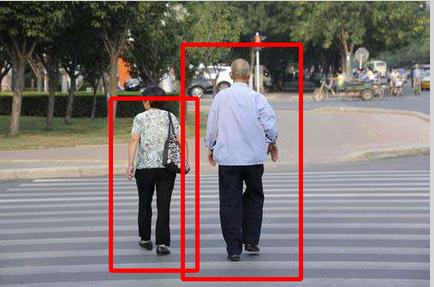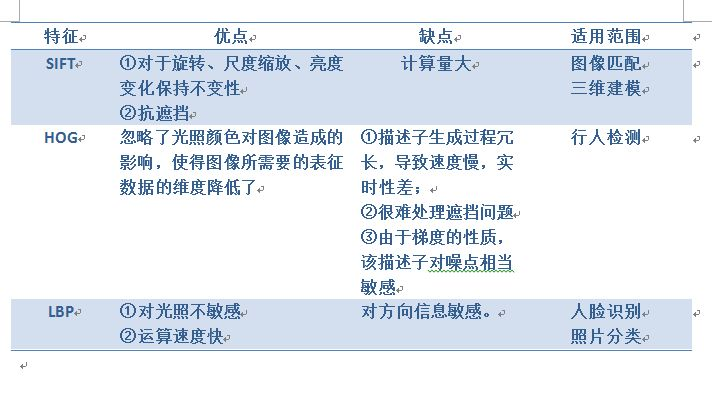# opencv LBP特征与行人识别

1、对图像中的所有点，以该点为中心，取3x3的邻域窗口；

2、将8-邻域像素值与中心点像素值进行比较，大于或等于中心像素标记为1，否则标记为0；

3、将周围0-1序列，以一定的顺序排列，成一个8位的无符号的二进制数，转化成整数；

4、这个整数就是表征这个窗口的LBP值。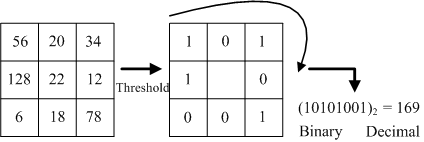1、产生的二进制模式多；

2、不具有旋转不变性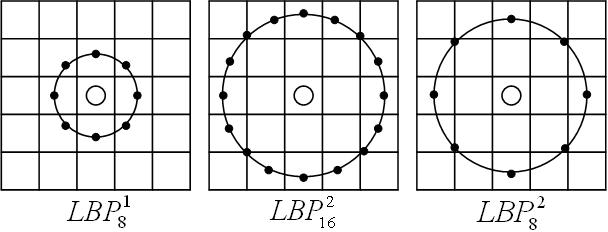Ojala提出一种“等价模式”（Uniform Pattern）来对LBP算子进行降维，Ojala等认为图像中，某个局部二进制模式所对应的循环二进制数从0—>1从1—>0，最多有两次跳变，该局部二进制模式所对应的二进制就成为一个等价模式。如00000000,00111000,10001111,11111111等都是等价模式类。判断一个二进制模式是否为等价模式最简单的办法就是将LBP值与其循环移动一位后的值进行按位相与，计算得到的二进制数中1的个数，若个数小于或等于2，则是等价模式；否则，不是。出了等价模式以外的模式都归一一类，称为混合模式类。

通过这种改进，二进制模式的种类大大减少，而不会丢失任何信息，模式种类由原来的2^p减少为p*(p-1)+2种。

但等价模式代表了图像的边缘、斑点、角点等关键模式，等价模式占了总模式中的绝大多数，所以极大的降低了特征维度。利用这些等价模式和混合模式类直方图，能够更好地提取图像的本质特征。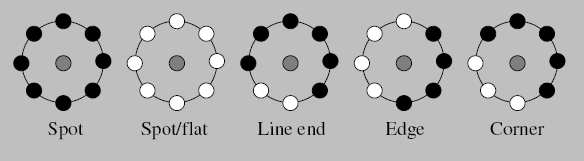## 旋转不变的LBP算子

由于LBP的二进制模式是以一定的方向、顺序进行编码的，所以当图像发生旋转时，按这种编码的话，LBP值会发生改变，因此是不具有旋转不变性的。Maenpaa等人提出了具有旋转不变性的LBP算子。

解决办法是：不断旋转邻域得到一系列的LBP值，取其中最小值作为该邻域的LBP值。旋转过程实质上就是对二进制模式进行循环移位的过程。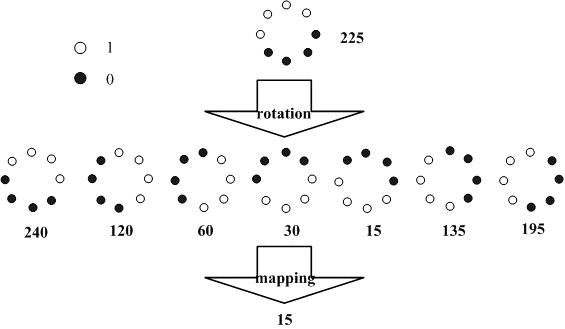LBP特征提取的demo:

void elbp(Mat& src, Mat &dst, int radius, int neighbors)
{

for (int n = 0; n<neighbors; n++)
{
// 采样点的计算
float x = static_cast<float>(-radius * sin(2.0*CV_PI*n / static_cast<float>(neighbors)));
float y = static_cast<float>(radius * cos(2.0*CV_PI*n / static_cast<float>(neighbors)));
// 上取整和下取整的值
int fx = static_cast<int>(floor(x));
int fy = static_cast<int>(floor(y));
int cx = static_cast<int>(ceil(x));
int cy = static_cast<int>(ceil(y));
// 小数部分
float ty = y - fy;
float tx = x - fx;
// 设置插值权重
float w1 = (1 - tx) * (1 - ty);
float w2 = tx  * (1 - ty);
float w3 = (1 - tx) *      ty;
float w4 = tx  *      ty;
// 循环处理图像数据
for (int i = radius; i < src.rows - radius; i++)
{
for (int j = radius; j < src.cols - radius; j++)
{
// 计算插值
float t = static_cast<float>(w1*src.at<uchar>(i + fy, j + fx) + w2*src.at<uchar>(i + fy, j + cx) + w3*src.at<uchar>(i + cy, j + fx) + w4*src.at<uchar>(i + cy, j + cx));
// 进行编码
dst.at<uchar>(i - radius, j - radius) += ((t > src.at<uchar>(i, j)) || (std::abs(t - src.at<uchar>(i, j)) < std::numeric_limits<float>::epsilon())) << n;
}
}
}
}

void elbp1(Mat& src, Mat &dst)
{

// 循环处理图像数据
for (int i = 1; i < src.rows - 1; i++)
{
for (int j = 1; j < src.cols - 1; j++)
{
uchar tt = 0;
int tt1 = 0;
uchar u = src.at<uchar>(i, j);
if (src.at<uchar>(i - 1, j - 1)>u) { tt += 1 << tt1; }
tt1++;
if (src.at<uchar>(i - 1, j)>u) { tt += 1 << tt1; }
tt1++;
if (src.at<uchar>(i - 1, j + 1)>u) { tt += 1 << tt1; }
tt1++;
if (src.at<uchar>(i, j + 1)>u) { tt += 1 << tt1; }
tt1++;
if (src.at<uchar>(i + 1, j + 1)>u) { tt += 1 << tt1; }
tt1++;
if (src.at<uchar>(i + 1, j)>u) { tt += 1 << tt1; }
tt1++;
if (src.at<uchar>(i + 1, j - 1)>u) { tt += 1 << tt1; }
tt1++;
if (src.at<uchar>(i - 1, j)>u) { tt += 1 << tt1; }
tt1++;

dst.at<uchar>(i - 1, j - 1) = tt;
}
}
}

int main()
{
Mat img = cv::imread("D:\\cv_study\\Exercise\\LBP\\lena.jpg", 0);
namedWindow("image");
imshow("image", img);

neighbors = 8;

//创建一个LBP
//注意为了溢出，我们行列都在原有图像上减去2个半径
Mat dst = Mat(img.rows - 2 * radius, img.cols - 2 * radius, CV_8UC1, Scalar(0));
elbp1(img, dst);
namedWindow("normal");
imshow("normal", dst);

Mat dst1 = Mat(img.rows - 2 * radius, img.cols - 2 * radius, CV_8UC1, Scalar(0));
elbp(img, dst1, 1, 8);
namedWindow("circle");
imshow("circle", dst1);

while (1)
waitKey(0);
}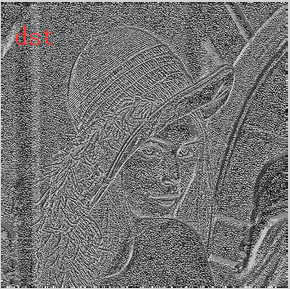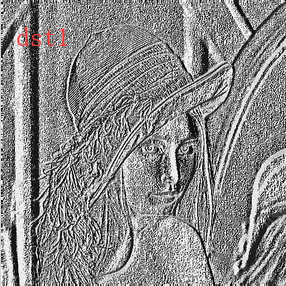int main()
{
Mat img = imread("D://cv_study//行人检测//person.jpg",1);
vector<Rect>found;
HOGDescriptor defaultHog;
imshow("原图", img);
defaultHog.setSVMDetector(HOGDescriptor::getDefaultPeopleDetector());
defaultHog.detectMultiScale(img, found, 0, Size(8, 8), Size(0, 0), 1.05, 2);
for (int i = 0; i < found.size(); i++)
{
Rect r = found[i];
rectangle(img,r.tl(),r.br(), Scalar(0, 0, 255), 3);
}
imshow("PEOPLE", img);
waitKey();
return 0;
}